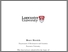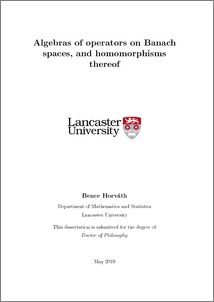# Algebras of operators on Banach spaces, and homomorphisms thereof

Horvath, Bence (2019) Algebras of operators on Banach spaces, and homomorphisms thereof. PhD thesis, UNSPECIFIED.Preview
PDF (2019horvathphd)
2019horvathphd.pdf - Published Version

## Abstract

This thesis concerns the theory of Banach algebras of operators on Banach spaces. The emphasis for most of the thesis is on the homomorphisms and perturbations of homomorphisms of such algebras. Chapter 2 of this thesis is devoted to the study of perturbations of homomorphisms between Banach algebras. We say that a bounded linear map ϕ : A → B between Banach algebras A and B is δ-multiplicative, if δ > 0 and ∥ϕ(ab)−ϕ(a)ϕ(b)∥ ≤ δ∥a∥∥b∥ for every a, b ∈ A. Intuitively, if ϕ is δ-multiplicative for a small δ then ϕ may be considered to be almost homomorphic. Another way of measuring how perturbed a bounded linear map is to see how near it is to the closed set of multiplicative bounded linear maps between A and B. One would like to explore, of course, the connection between these two notions. If a bounded linear map is ϵ-close to a genuine homomorphism in norm, then it is δ-multiplicative for some δ depending on ϵ and the norm of the map. More precisely: Let A, B be Banach algebras and let ϵ, K > 0. It is easy to check (see [38, Proposition 1.1]) that there is a δ > 0 such that for any bounded linear map ϕ : A → B with ∥ϕ∥ ≤ K, if there is a bounded linear multiplicative map ψ : A → B with ∥ϕ − ψ∥ < δ, then ϕ is ϵ-multiplicative. The converse direction is far from being true, in general. Pairs of Banach algebras (A, B) which satisfy the converse direction, are said to have the AMNM property, see Definition 2.1.1 for a precise formulation. Although this topic has its roots in the classical Hyers–Ulam stability theory, it was really K. Jarosz who initiated the study of this field in . Later, B. E. Johnson in  studied the AMNM property of Banach algebras of the form (A, K), where A is a commutative Banach algebra over some scalar field K. He substantially extended the scope of his study in his seminal paper , where he now turned his attention to general pairs of Banach algebras. Arguably, the most important result of his paper is [38, Theorem 3.1], which states that if A is an amenable Banach algebra and B is a dual Banach algebra then (A, B) has the AMNM property. By carefully studying the proof of Johnson’s theorem, we extend this result in Theorem 2.3.3. This allows us to prove the main result (Theorem 2.1.2) of Chapter 2, namely, that (B(E), B(F)) has the AMNM property whenever E ∈ {ℓ1, Lp[0, 1] : 1 ≤ p < ∞} or E is a Banach space with a subsymmetric, shrinking Schauder basis, and F is a separable, reflexive Banach space. (Here B(E) denotes the Banach algebra of bounded linear operators on E.) This extends another result of Johnson; it was shown in [38, Proposition 6.3] that (B(H), B(H)) has the AMNM property, where H is a separable Hilbert space. We would like to draw the reader’s attention to the fact that rather non-trivial methods are needed to obtain this latter result. The reason behind this is twofold: Firstly, B(E) is very rarely amenable for infinite-dimensional Banach spaces E, see . Secondly, when considering bounded linear maps between B(E) and B(F), they are not assumed to be weak∗ - to - weak∗ continuous, even if both E and F are reflexive. In the penultimate section of Chapter 2 we study δ-multiplicative maps φ : A(E) → B(F), where E and F are Banach spaces and A(E) denotes the Banach algebra of approximable operators on E. Inspired by a result of M. D. P. Daws ([17, Lemma 3.3.14]), we show that if E has a Schauder basis, φ is a δ-multiplicative, norm one linear map from A(E) to B(F) for a sufficiently small δ > 0, then F has a closed subspace isomorphic to E. In the last section of Chapter 2 we establish a connection between the second bounded Hochschild cohomology group of a Banach algebra A with coefficients in a Banach A-bimodule X, and the AMNM property of the pair (A, A ⋉ X), where A ⋉ X denotes the semi-direct product of A with X. In Chapter 3 we study representations of Banach algebras of the form B(E). More precisely, we are interested in the following question: Suppose E is a Banach space. If F is a non-zero Banach space and ψ : B(E) → B(F) is a surjective algebra homomorphism, is ψ necessarily injective? Positive answer to this question would imply — by a classical result of Eidelheit, see e.g. [15, Theorem 2.5.7] — that E and F are isomorphic. If E satisfies the property above for every non-zero Banach space F and every surjective algebra homomorphism ψ : B(E) → B(F), then we say that E has the SHAI property (Definition 3.1.1). As we shall see in Chapter 3, many of the classical Banach spaces and Hilbert spaces of arbitrary density character (that is, of arbitrarily large dimension) satisfy this property (see Example 3.3.8 and Theorem 3.4.8), as well as some more exotic ones (Corollary 3.3.12 and Theorem 3.4.6). We demonstrate in Proposition 3.5.3 that the SHAI property is stable under taking finite sums. We also give many examples for Banach spaces which fail to have this property, see Examples 3.2.2. In the last section of Chapter 3, we study the question in the “opposite direction”: Can we find infinite-dimensional Banach spaces E and F and a surjective algebra homomorphism ψ : B(E) → B(F) which is not injective? We shall see in Theorem 3.6.13 that for every separable, reflexive Banach space F it is possible to find a Banach space E and a surjective algebra homomorphism ψ : B(E) → B(F) which is not injective. In Chapter 4 we study certain ring-theoretic properties of B(E), characterised by their idempotents. In the classical (non-commutative) ring-theoretic context the notions of Dedekind-finiteness, Dedekind-infiniteness and proper infiniteness are very well know. In a Banach algebraic context, there is a stronger notion than Dedekindfiniteness; this is having stable rank one, in the sense of Rieffel, see Definition 4.1.3 and Lemma 4.2.1. The study of these ring-theoretic properties of B(E) was laid out by N. J. Laustsen in . In particular, it was shown in the aforementioned paper that B(E) is Dedekind-finite whenever E is a complex hereditarily indecomposable Banach space. We shall see in Corollary 4.2.9 that in fact more is true, for such a Banach space E, its algebra of operators B(E) has stable rank one. To complement this, we show in Theorems 4.2.16 and 4.3.5 that there are examples for both complex and real Banach spaces such that their algebras of operators are Dedekind-finite but they do not have stable rank one. The complex example is provided by the complexification of Tarbard’s indecomposable but not hereditarily indecomposable space X∞, and the real example is C(K, R), where K is a connected Koszmider space. In the last section of Chapter 4 we explore the connection between the existence of a certain unique maximal ideal in a Banach algebra and Dedekind-finiteness.

Item Type:
Thesis (PhD)
Departments:
ID Code:
133919
Deposited By:
Deposited On:
25 Jun 2019 09:30
Refereed?:
No
Published?:
Published